## Thursday, June 30, 2011

### 106

106 = 2 x 53.

106 has a representation as a sum of two squares: 106 = 52 + 92.

106 is a divisor of 234 - 1.

106 = 25 + 26 + 27 + 28.

15 straight cuts through a pizza can produce as many as 106 pieces.

106 is a centered pentagonal number and a centered heptagonal number.

The sum of the first 106 digits of pi is a prime.Messier 106 is a bright spiral galaxy in the constellation Canes Venatici.

## Wednesday, June 29, 2011

### 8880

8880 = 24 x 3 x 5 x 37.

8880 is a divisor of 314 - 1.

8880 is 3344 in base 14.

8880 is a number that can be expressed as the difference of the squares of primes in just three distinct ways.

8880 is a multiple of 24 whose digits also sum to 24.The 8880 Super Car was a Lego Technic kit released in 1994.

## Tuesday, June 28, 2011

### 837

837 = 33 x 31.

837 is a divisor of 266 - 1.

837 is 345 in base 16.

837 is the sum of two consecutive primes and the composite numbers in between: 837 = 89 + 90 + 91 + 92 + 93 + 94 + 95 + 96 + 97.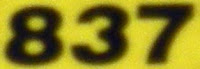Halley's comet was particularly bright when it appeared in the year 837 and made one of its closest approaches to Earth.

## Monday, June 27, 2011

### 464

464 = 24 x 29.

464 has a representation as a sum of two squares: 464 = 82 + 202.

464 is the maximum number of regions into which 22 circles can divide the plane. It is also the maximum number of volumes into which 12 spheres can divide space.

464 is 565 in base 9. It is 252 in base 14.In chess, 464 is the number of legal positions of the kings, not counting mirrored positions.

## Friday, June 24, 2011

### 702

702 = 2 x 33 x 13.

702 is a pronic number because it is the product of two consecutive integers: 702 = 26 x 27.

702 is 222000 in base 3 and 2022 in base 7.

702 is a number n such that n3 is the sum of two consecutive primes: 7023 = 345,948,408 = 172,974,199 + 172,974,209.702 is a musical group (R&B trio) named after the area code of their hometown: Las Vegas, Nevada.

## Thursday, June 23, 2011

### 159

159 = 3 x 53. Concatenating the prime factors gives 353, which is a peak palindrome and a prime number.

In 1973, Jean-Marc Deshouillers showed that all integers are the sum of at most 159 prime numbers.

159 is a number that cannot be written as a sum of three squares.

159 x 48 = 7632 uses all the digits from 1 to 9 once.

1352, 1592, and 2852 use the same digits: 1352 = 18225; 1592 = 25281; and 2852 = 81,225.The chemical element terbium has one stable isotope, which has as mass number of 159.

## Wednesday, June 22, 2011

### 8810

8810 = 2 x 5 x 881.

8810 has two representations as a sum of two squares: 8810 = 232 + 912 = 592 + 732.

8810 has a square whose digits each occur twice: 88102 = 77,616,100.

8810 is a number n such that n time n + 7 gives the concatenation of two numbers m and m + 3: 8810 x 8817 = 77,677,770.The Nokia 8810 was a mobile phone introduced in 1998 and available only in Europe.

## Tuesday, June 21, 2011

### 880

880 = 24 x 5 x 11.

880 is the number of 4 x 4 magic squares.

880 is a number that can't be represented as a sum of three squares.

880 is 1177 in base 9.880 Herba is an asteroid discovered in 1917.

## Monday, June 20, 2011

### 5700

5700 = 22 x 3 x 52 x 19.

5700 is a pronic number because it is the product of two consecutive numbers: 5700 = 75 x 76.

5700 is divisible by its reverse (75).

5700 is 22422 in base 7 and 2112 in base 14. It is 7733 in base 9. 5700 in base 3 (21211010) and in base 4 (1121010) ends with the same four digits: 1010.The Nokia 5700 XpressMusic is a mobile phone that emphasizes music and multimedia playback.

## Friday, June 17, 2011

### 580

580 = 22 x 5 x 29.

580 is 1001000100 in base 2 (binary). The base 3 (210111) and base 4 (21010) representations of 580 both start with 2101. 580 is 2404 in base 6 and 404 in base 12. It is 202 in base 17 and 104 in base 24.

580 has two representations as a sum of two squares: 580 = 22 + 242 = 162 + 182.

580 is the sum of six consecutive primes: 580 = 83 + 89 + 97 + 101 + 103 + 107.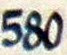Telephone area code 580 serves the state of Oklahoma.

## Thursday, June 16, 2011

### 148

148 = 22 x 37.

148 has a representation as a sum of two squares: 148 = 22 + 122.

148 is a heptagonal number. It is also a centered heptagonal number.

148 is the number of perfect graphs with six vertices.

148 x (14 + 8) = 143 + 83 = 3256.

Three square numbers using the same digits: 1482 = 21904; 2032 = 41209; and 3022 = 91204.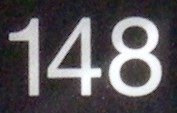The astronomical object Arp 148 is the spectacular aftermath of an encounter between two galaxies.

Source: Numeropedia

## Wednesday, June 15, 2011

### 381

381 = 3 x 127.

381 is 101111101 in base 2 (binary). It is 575 in base 8 and 111 in base 19.

381 is the sum of the first 16 primes: 381 = 2 + 3 + 5 + 7 + 11 + 13 + 17 + 19 + 23 + 29 + 31 + 37 + 41 + 43 + 47 + 53.

The sum of the divisors of 381 is a cube: 1 + 3 + 127 + 381 = 512 = 83.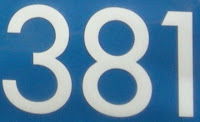381 can represent the term "I Love You" (three words, eight letters, one meaning).

Source: Wikipedia

## Tuesday, June 14, 2011

### 653

653 is a prime number. It is a Sophie Germain prime because 2 x 653 + 1 = 1307 is a prime.

653 is the first three-digit prime number to occur in the decimal expansion of pi.

653 has a representation as a sum of two squares: 653 = 132 + 222.

653 is the hypotenuse of a primitive Pythagorean triple: 6532 = 3152 + 5722.

653 is the sum of the squares of five consecutive primes: 653 = 52 + 72 + 112 + 132 + 172.ARINC 653 is a software specification for space and time partitioning in safety-critical avionics real-time operating systems.

## Monday, June 13, 2011

### 1424

1424 = 24 x 89.

1424 has a representation as a sum of two squares: 1424 = 202 + 322.

1424 is a number for which the sum of the reciprocals of the digits is an integer: 1/1 + 1/4 + 1/2 + 1/4 = 2.

1424 is the sum of four squares in exactly seven ways. It is the sum of four distinct squares in exactly two ways.

1424 uses only the digits 1, 2, and/or 4 in base 4 (112100), base 5 (21144), and base 10 (1424).Hotel 1424 is in St. Helena, California.

## Friday, June 10, 2011

### 6287

6287 is a prime number.

6287 is a number that cannot be written as a sum of three squares.

6287 is the central term of five successive primes such that their average is a prime.

6287 is an odious number because it has an odd number of 1s in its binary expansion: 22121212.NGC 6287 is a globular cluster in the constellation Ophiuchus.

## Thursday, June 9, 2011

### 644

644 = 22 x 7 x 23.

644 is a Perrin number.

644 is 212212 in base 3 and 2552 in base 6.

644 is a number made up of only semiprime digits (4, 6, and 9).

644 is the sum of five fourth powers: 644 = 54 + 24 + 14 + 14 + 14.The USS Lewis and Clark (SSBN-644) was a ballistic missile submarine in the U.S. Navy.

## Wednesday, June 8, 2011

### 2098

2098 = 2 x 1049. It is a semiprime with a prime sum of factors: 2 + 1049 = 1051, a prime. It is also a semiprime one less than a prime: 2098 + 1 = 2099, a prime. The sum of its digits is a prime: 2 + 0 + 9 + 8 = 19, a prime.

2098 has a representation as a sum of two squares: 2098 = 272 + 372.

2098 is 747 in base 17.

2098 is the sum of 12 sixth powers.A transit of Mercury (its passage across the sun) is expected to occur in the year 2098.

## Tuesday, June 7, 2011

### 1100

1100 = 22 x 52 x 11.

1100 has a base 2 (binary) representation that ends in the digits 1100: 10001001100. 1100 is 3131 in base 7. It is 778 in base 12, 668 in base 13, and 588 in base 14.

1100 is the sum of two palindromic primes in three ways: 1100 = 181 + 919 = 313 + 787 = 373 + 727.

1100 is the sum of a square and a cube: 1100 = 103 + 102. It is the sum of two distinct powers of 10.

1100 is the sum of eight positive fifth powers: 1100 = 35 + 35 + 35 + 35 + 25 + 25 + 25 + 25.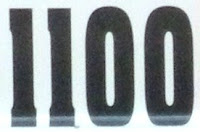1100 AD is an online multiplayer strategy games set in medieval times.

## Monday, June 6, 2011

### 757

757 is a prime number.

757 has a representation as a sum of two squares: 757 = 92 + 262.

757 is the hypotenuse of a primitive Pythagorean triple: 7572 = 4682 + 5952.

757 is the smallest palindromic prime that remains prime when its leading digit is replaced by any nonzero digit that is a power of 2: 157, 257, 457, 857.

757 is the largest of three three-digit palindromic primes that are not the sum of consecutive composite numbers. The others are 313 and 383.The Boeing 757 is a mid-size, narrow-body, twin-engine jetliner.

## Friday, June 3, 2011

### 879

879 = 3 x 293.

879 is a number that cannot be written as a sum of three squares.

The fifth root of 879 has a decimal part that begins with the digits 879: 3.879696696. . .

The sum of the digits of 879 is 8 times the number of digits: 8 + 7 + 9 = 24 = 8 x 3.

879 is a number n such that n - 2 and n + 2 are primes: 877 and 881.NGC 879 is a galaxy in the constellation Cetus, discovered in 1886.

## Thursday, June 2, 2011

### 536

536 = 23 x 67.

536 starts with the same four digits in base 3 and base 4: 201212 and 20120. It has double digits in base 6 (2252), base 9 (655), base 11 (448), and base 12 (388). It is 323 in base 13.

536 is the lowest happy number beginning with the digit 5.

536 is the solution to the Dancing School Problem with 3 girls and 12 boys.The stomachion of Archimedes (a 14-piece dissection puzzle) has 536 solutions.

## Wednesday, June 1, 2011

### 450

450 = 2 x 32 x 52.

450 has two representations as a sum of two squares: 450 = 32 + 212 = 152 + 152.

450 is 3300 in base 5, 1212 in base 7, 550 in base 9, 242 in base 14, and 200 in base 15.

450 is the sum of two consecutive primes: 450 = 223 + 227.

450 and 4502 = 202,500 have the same digit sum: 4 + 5 + 0 = 2 + 0 + 2 + 5 + 0 + 0 = 9.450 is a perfect score in Canadian five-pin bowling.